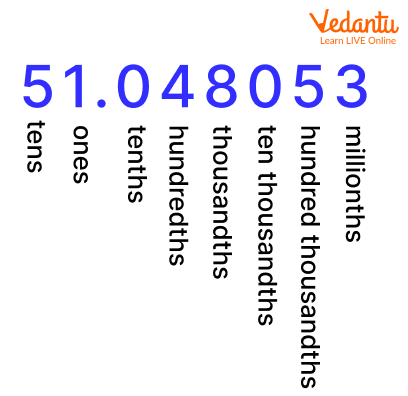Courses
Courses for Kids
Free study material
Free LIVE classes
More

# Round Off a Number to the Nearest HundredthLIVE
Join Vedantu’s FREE Mastercalss

## What is the Concept Behind Rounding-off?

The concept of rounding-off was developed to get the approximate value of numbers in decimal because any number like 23.1345 would look odd but when we can make it simpler to 23.13 i.e., to the nearest hundredth value. Thus, rounding off makes the long decimal numbers easier to remember.

We stated that rounding-off is similar to approximation and we represent it by a symbol ≈, which is a short way to display the round-off of any number because the math works more on symbols than prolonged statements.

Now, let us get through the process of finding the round-off of a number to the nearest hundredth with solved examples.

### Process to Round-off to the Nearest Hundredth

In Maths, the decimal numbers are the numbers that rely on the powers of 10, which means when we move from left to right, the place value of the number gets divided by 10.

Hence, the place value system is the best method to determine the tenths, hundredths, thousandths, and so on of the digits of any given number.

Here, tenth means 1/10, hundredth means 1/100, thousandth means 1/1000, and so on.

### What is the Hundredth of a Number?

The image you can see below clearly shows the place value of a decimal number.Representation of the hundredth place of a decimal number?

So, the digits to the left are 5 and 1, where 1 is at the one’s place and 5 is at the tens place, which means from right to left, the place value increases. Whereas going from left to right after the decimal point, we notice that the place value of ‘0’ is at tenths place or1/10, 4 at hundredths place or 4/100, 8 is at thousandths place or 8/1000, and so on.

While rounding the number to its nearest hundredth place, we must follow the given steps:

Let us assume a decimal number: 3.1736

Now, we have to round off the number 3. 1736 to its nearest hundredth value.

Step 1: Firstly, we need to recognise the hundredth digit. (Here, 7 is the digit in the hundredth position or it has the hundredth place value)

Step 2: Now, we proceed with the digit that is just next to the hundredth digit. (Here, 3 is the digit, which is just after the hundredth digit)

Step 3: Now, we need to check whether the digit next to the hundredth place is greater than or equal to 5, round up the hundredth digit, or else round down the hundredth digit. (Here, we notice that 3 is less than 5, so we round down).

Step 4: Since the digit after the hundredth place was lesser than 5 so the digit at the hundredth place remains the same as 6, as 2 is less than 5.

Hence, the number 3.1736 rounds off to its nearest hundredth as 3.17.

### Examples of Rounding off to the Nearest Hundredth

• 6. 1234

Here, 2 is the digit at the hundredth place and the digit just after it is ‘3’ which is lesser than 5, so the 6.1234 rounds off to 6.12

• 5.1289

Here, 2 is at the hundredth place and 8 is at the thousandth place which is greater than 5, so here we have a new case when 8 > 5 and ‘2’ at the hundredth place changes to ‘3’. Hence, the round-off to the nearest hundredth becomes 5.13 for 5.1289.

### A Fact to Remember:

Approximately equal to sign is often used to indicate rounding of exact numbers, e.g., 9.98 ≈ 10. This sign was introduced by Alfred George Greenhill in 1892.

So, this was all about the round-off concept. The above context explains to us that if the digit at the thousandth place is lesser than 5 then we just take two places after the decimal as such (we did in an example 3.1736). However, when the same digit is greater than 5, we increase the value of the digit at the hundredths place by 1, as we did in the example of 5.1289.

Last updated date: 16th Sep 2023
Total views: 136.5k
Views today: 4.36k

## FAQs on Round Off a Number to the Nearest Hundredth

1. Is a decimal also an integer?

We can express any integer in a decimal form. However, the numbers that we express as a decimal are not generally integers. Also, whenever a number has zero after the decimal point is zero (0), it is surely an integer.

2. What are decimal numbers?

We use the decimal numbers for representing the whole numbers on the left side of the decimal (a period) and the fractional parts that are lesser than 1 unit, written at the right side of the decimal. In the nutshell, decimals are the shorthand method for writing the fractions and mixed numbers with denominators that usually are the powers of 10, such as 10, 100, 1000, etc.